# 貌离神合的RNN与ODE：花式RNN简介

2018-6-29 14:15| 发布者: 炼数成金_小数| 查看: 16528| 评论: 0|原作者: 苏剑林|来自: PaperWeekly网络工具模型数学时间序列函数 作者丨苏剑林单位丨广州火焰信息科技有限公司研究方向丨NLP，神经网络个人主页丨kexue.fm本来笔者已经决心不玩 RNN 了，但是在上个星期思考时忽然意识到 RNN 实际上对应了 ODE（常微分方程）的数值解法，这为我一直以来想做的事情——用深度学习来解决一些纯数学问题——提供了思路。事实上这是一个颇为有趣和有用的结果，遂介绍一翻。顺便地，本文也涉及到了自己动手编写 RNN 的内容，所以本文也可以作为编写自定义的 RNN 层的一个简单教程。注：本文并非前段时间的热点“神经 ODE ”的介绍（但有一定的联系）。RNN基本什么是RNN？ 众所周知，RNN 是“循环神经网络（Recurrent Neural Network）”，跟 CNN 不同，RNN 可以说是一类模型的总称，而并非单个模型。简单来讲，只要是输入向量序列 (x1,x2,…,xT)，输出另外一个向量序列 (y1,y2,…,yT)，并且满足如下递归关系的模型，都可以称为 RNN。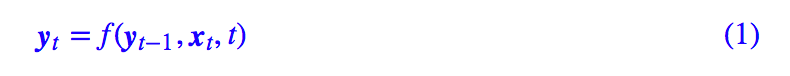也正因为如此，原始的朴素 RNN，还有改进的如 GRU、LSTM、SRU 等模型，我们都称为 RNN，因为它们都可以作为上式的一个特例。还有一些看上去与 RNN 没关的内容，比如前不久介绍的 CRF 的分母的计算，实际上也是一个简单的 RNN。说白了，RNN 其实就是递归计算。自己编写RNN这里我们先介绍如何用 Keras 简单快捷地编写自定义的 RNN。 事实上，不管在 Keras 还是纯 tensorflow 中，要自定义自己的 RNN 都不算复杂。在 Keras 中，只要写出每一步的递归函数；而在 tensorflow 中，则稍微复杂一点，需要将每一步的递归函数封装为一个 RNNCell 类。下面介绍用 Keras 实现最基本的一个 RNN：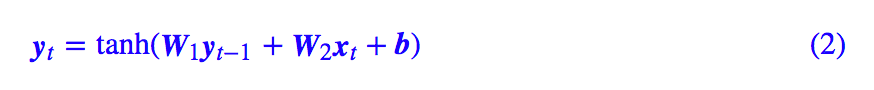代码非常简单：#! -*- coding: utf-8- -*-from keras.layers import Layerimport keras.backend as Kclass My_RNN(Layer):    def __init__(self, output_dim, **kwargs):        self.output_dim = output_dim # 输出维度        super(My_RNN, self).__init__(**kwargs)    def build(self, input_shape): # 定义可训练参数        self.kernel1 = self.add_weight(name='kernel1',                                      shape=(self.output_dim, self.output_dim),                                      initializer='glorot_normal',                                      trainable=True)        self.kernel2 = self.add_weight(name='kernel2',                                      shape=(input_shape[-1], self.output_dim),                                      initializer='glorot_normal',                                      trainable=True)        self.bias = self.add_weight(name='kernel',                                      shape=(self.output_dim,),                                      initializer='glorot_normal',                                      trainable=True)    def step_do(self, step_in, states): # 定义每一步的迭代        step_out = K.tanh(K.dot(states, self.kernel1) +                          K.dot(step_in, self.kernel2) +                          self.bias)        return step_out, [step_out]    def call(self, inputs): # 定义正式执行的函数        init_states = [K.zeros((K.shape(inputs),                                self.output_dim)                              )] # 定义初始态(全零)        outputs = K.rnn(self.step_do, inputs, init_states) # 循环执行step_do函数        return outputs # outputs是一个tuple，outputs为最后时刻的输出，                          # outputs为整个输出的时间序列，output是一个list，                          # 是中间的隐藏状态。    def compute_output_shape(self, input_shape):        return (input_shape, self.output_dim)可以看到，虽然代码行数不少，但大部分都只是固定格式的语句，真正定义 RNN 的，是 step_do 这个函数，这个函数接受两个输入：step_in 和 states。其中 step_in 是一个 (batch_size, input_dim) 的张量，代表当前时刻的样本 xt，而 states 是一个 list，代表 yt−1 及一些中间变量。特别要注意的是，states 是一个张量的 list，而不是单个张量，这是因为在递归过程中可能要同时传递多个中间变量，而不仅仅是 yt−1 一个，比如 LSTM 就需要有两个态张量。最后 step_do 要返回 yt 和新的 states，这是 step_do 这步的函数的编写规范。 而 K.rnn 这个函数，接受三个基本参数（还有其他参数，请自行看官方文档），其中第一个参数就是刚才写好的 step_do 函数，第二个参数则是输入的时间序列，第三个是初始态，跟前面说的 states 一致，所以很自然 init_states 也是一个张量的 list，默认情况下我们会选择全零初始化。ODE基本什么是ODE？ODE 就是“常微分方程（Ordinary Differential Equation）”，这里指的是一般的常微分方程组：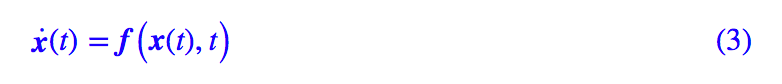研究 ODE 的领域通常也直接称为“动力学”、“动力系统”，这是因为牛顿力学通常也就只是一组 ODE 而已。ODE可以产生非常丰富的函数。比如 e^t 其实就是 x˙=x 的解，sint 和 cost 都是 x¨+x=0 的解（初始条件不同）。事实上，我记得确实有一些教程是直接通过微分方程 x˙=x 来定义 e^t 函数的。除了这些初等函数，很多我们能叫得上名字但不知道是什么鬼的特殊函数，都是通过 ODE 导出来的，比如超几何函数、勒让德函数、贝塞尔函数...总之，ODE 能产生并且已经产生了各种各样千奇百怪的函数～数值解ODE 能较精确求出解析解的 ODE 其实是非常少的，所以很多时候我们都需要数值解法。 ODE 的数值解已经是一门非常成熟的学科了，这里我们也不多做介绍，仅引入最基本的由数学家欧拉提出来的迭代公式：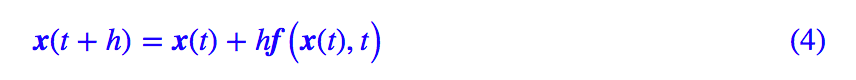这里的 h 是步长。欧拉的解法来源很简单，就是用：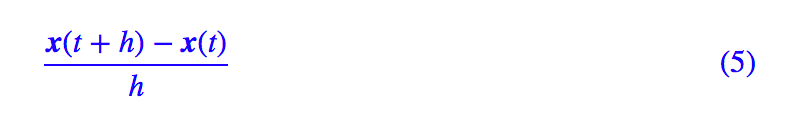来近似导数项 x˙(t)。只要给定初始条件 x(0)，我们就可以根据 (4) 一步步迭代算出每个时间点的结果。ODE与RNN ODE也是RNN大家仔细对比 (4) 和 (1)，发现有什么联系了吗？在 (1) 中，t 是一个整数变量，在 (4) 中，t 是一个浮点变量，除此之外，(4) 跟 (1) 貌似就没有什么明显的区别了。事实上，在 (4) 中我们可以以 h 为时间单位，记 t=nh，那么 (4) 变成了：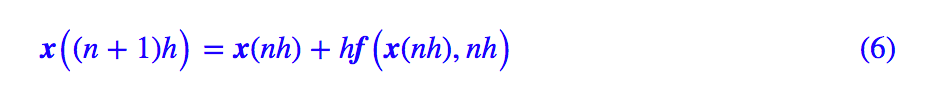可以看到现在 (6) 中的时间变量 n 也是整数了。这样一来，我们就知道：ODE 的欧拉解法 (4) 实际上就是 RNN 的一个特例罢了。这里我们也许可以间接明白为什么 RNN 的拟合能力如此之强了（尤其是对于时间序列数据），我们看到 ODE 可以产生很多复杂的函数，而 ODE 只不过是 RNN 的一个特例罢了，所以 RNN 也就可以产生更为复杂的函数了。 用RNN解ODE 于是，我们就可以写一个 RNN 来解 ODE 了，比如《两生物种群竞争模型》 中的例子：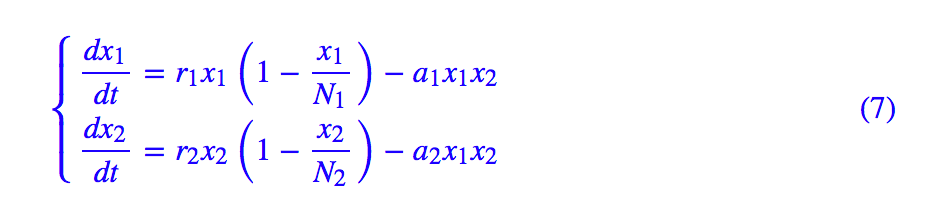我们可以写出：#! -*- coding: utf-8- -*-from keras.layers import Layerimport keras.backend as Kclass ODE_RNN(Layer):    def __init__(self, steps, h, **kwargs):        self.steps = steps        self.h = h        super(ODE_RNN, self).__init__(**kwargs)    def step_do(self, step_in, states): # 定义每一步的迭代        x = states        r1,r2,a1,a2,iN1,iN2 = 0.1,0.3,0.0001,0.0002,0.002,0.003        _1 = r1 * x[:,0] * (1 - iN1 * x[:,0]) - a1 * x[:,0] * x[:,1]        _2 = r2 * x[:,1] * (1 - iN2 * x[:,1]) - a2 * x[:,0] * x[:,1]        _1 = K.expand_dims(_1, 1)        _2 = K.expand_dims(_2, 1)        _ = K.concatenate([_1, _2], 1)        step_out = x + self.h * _        return step_out, [step_out]    def call(self, inputs): # 这里的inputs就是初始条件        init_states = [inputs]        zeros = K.zeros((K.shape(inputs),                         self.steps,                         K.shape(inputs))) # 迭代过程用不着外部输入，所以                                              # 指定一个全零输入，只为形式上的传入        outputs = K.rnn(self.step_do, zeros, init_states) # 循环执行step_do函数        return outputs # 这次我们输出整个结果序列    def compute_output_shape(self, input_shape):        return (input_shape, self.steps, input_shape)from keras.models import Sequentialimport numpy as npimport matplotlib.pyplot as pltsteps,h = 1000,0.1M = Sequential()M.add(ODE_RNN(steps, h, input_shape=(2,)))M.summary()# 直接前向传播就输出解了result = M.predict(np.array([[100, 150]])) # 以[100, 150]为初始条件进行演算times = np.arange(1, steps+1) * h# 绘图plt.plot(times, result[:,0])plt.plot(times, result[:,1])plt.savefig('test.png')整个过程很容易理解，只不过有两点需要指出一下。首先，由于方程组 (7) 只有两维，而且不容易写成矩阵运算，因此我在 step_do 函数中是直接逐位操作的（代码中的 x[:,0],x[:,1]），如果方程本身维度较高，而且能写成矩阵运算，那么直接利用矩阵运算写会更加高效；然后，我们可以看到，写完整个模型之后，直接 predict 就输出结果了，不需要“训练”。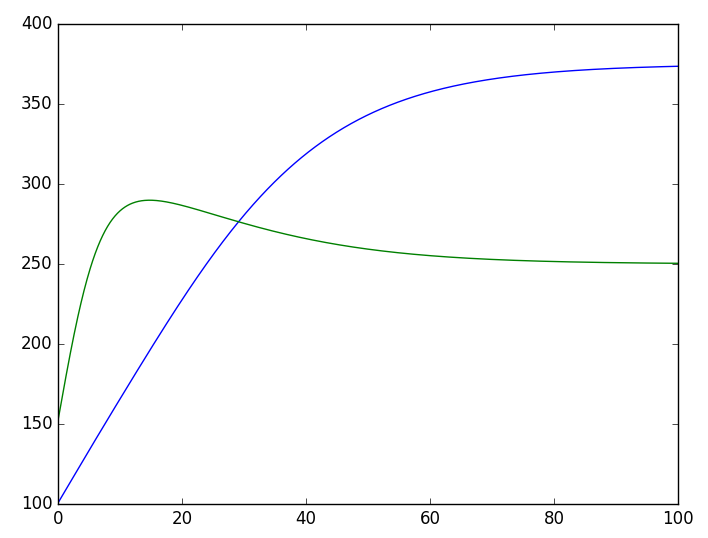RNN解两物种的竞争模型反推ODE参数前一节的介绍也就是说，RNN 的前向传播跟 ODE 的欧拉解法是对应的，那么反向传播又对应什么呢？在实际问题中，有一类问题称为“模型推断”，它是在已知实验数据的基础上，猜测这批数据符合的模型（机理推断）。这类问题的做法大概分两步，第一步是猜测模型的形式，第二步是确定模型的参数。假定这批数据可以由一个 ODE 描述，并且这个 ODE 的形式已经知道了，那么就需要估计里边的参数。如果能够用公式完全解出这个 ODE，那么这就只是一个非常简单的回归问题罢了。但前面已经说过，多数 ODE 都没有公式解，所以数值方法就必须了。这其实就是 ODE 对应的 RNN 的反向传播所要做的事情：前向传播就是解 ODE（RNN 的预测过程），反向传播自然就是推断 ODE 的参数了（RNN 的训练过程）。这是一个非常有趣的事实：ODE 的参数推断是一个被研究得很充分的课题，然而在深度学习这里，只是 RNN 的一个最基本的应用罢了。我们把刚才的例子的微分方程的解数据保存下来，然后只取几个点，看看能不能反推原来的微分方程出来，解数据为：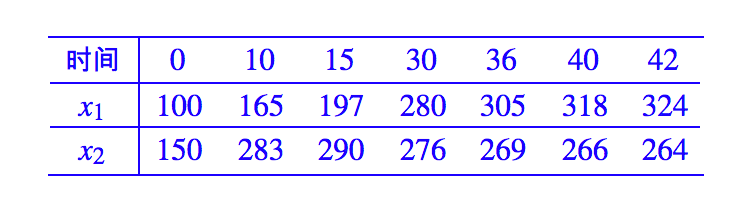假设就已知这有限的点数据，然后假定方程 (7) 的形式，求方程的各个参数。我们修改一下前面的代码：#! -*- coding: utf-8- -*-from keras.layers import Layerimport keras.backend as Kdef my_init(shape, dtype=None): # 需要定义好初始化，这相当于需要实验估计参数的量级    return K.variable([0.1, 0.1, 0.001, 0.001, 0.001, 0.001])class ODE_RNN(Layer):    def __init__(self, steps, h, **kwargs):        self.steps = steps        self.h = h        super(ODE_RNN, self).__init__(**kwargs)    def build(self, input_shape): # 将原来的参数设为可训练的参数        self.kernel = self.add_weight(name='kernel',                                       shape=(6,),                                      initializer=my_init,                                      trainable=True)    def step_do(self, step_in, states): # 定义每一步的迭代        x = states        r1,r2,a1,a2,iN1,iN2 = (self.kernel, self.kernel,                               self.kernel, self.kernel,                               self.kernel, self.kernel)        _1 = r1 * x[:,0] * (1 - iN1 * x[:,0]) - a1 * x[:,0] * x[:,1]        _2 = r2 * x[:,1] * (1 - iN2 * x[:,1]) - a2 * x[:,0] * x[:,1]        _1 = K.expand_dims(_1, 1)        _2 = K.expand_dims(_2, 1)        _ = K.concatenate([_1, _2], 1)        step_out = x + self.h * K.clip(_, -1e5, 1e5) # 防止梯度爆炸        return step_out, [step_out]    def call(self, inputs): # 这里的inputs就是初始条件        init_states = [inputs]        zeros = K.zeros((K.shape(inputs),                         self.steps,                         K.shape(inputs))) # 迭代过程用不着外部输入，所以                                              # 指定一个全零输入，只为形式上的传入        outputs = K.rnn(self.step_do, zeros, init_states) # 循环执行step_do函数        return outputs # 这次我们输出整个结果序列    def compute_output_shape(self, input_shape):        return (input_shape, self.steps, input_shape)from keras.models import Sequentialfrom keras.optimizers import Adamimport numpy as npimport matplotlib.pyplot as pltsteps,h = 50, 1 # 用大步长，减少步数，削弱长时依赖，也加快推断速度series = {0: [100, 150],          10: [165, 283],          15: [197, 290],          30: [280, 276],          36: [305, 269],          40: [318, 266],          42: [324, 264]}M = Sequential()M.add(ODE_RNN(steps, h, input_shape=(2,)))M.summary()# 构建训练样本# 其实就只有一个样本序列，X为初始条件，Y为后续时间序列X = np.array([series])Y = np.zeros((1, steps, 2))for i,j in series.items():    if i != 0:        Y[0, int(i/h)-1] += series[i]# 自定义loss# 在训练的时候，只考虑有数据的几个时刻，没有数据的时刻被忽略def ode_loss(y_true, y_pred):    T = K.sum(K.abs(y_true), 2, keepdims=True)    T = K.cast(K.greater(T, 1e-3), 'float32')    return K.sum(T * K.square(y_true - y_pred), [1, 2])M.compile(loss=ode_loss,          optimizer=Adam(1e-4))M.fit(X, Y, epochs=10000) # 用低学习率训练足够多轮# 用训练出来的模型重新预测，绘图，比较结果result = M.predict(np.array([[100, 150]]))times = np.arange(1, steps+1) * hplt.clf()plt.plot(times, result[:,0], color='blue')plt.plot(times, result[:,1], color='green')plt.plot(series.keys(), [i for i in series.values()], 'o', color='blue')plt.plot(series.keys(), [i for i in series.values()], 'o', color='green')plt.savefig('test.png')结果可以用一张图来看：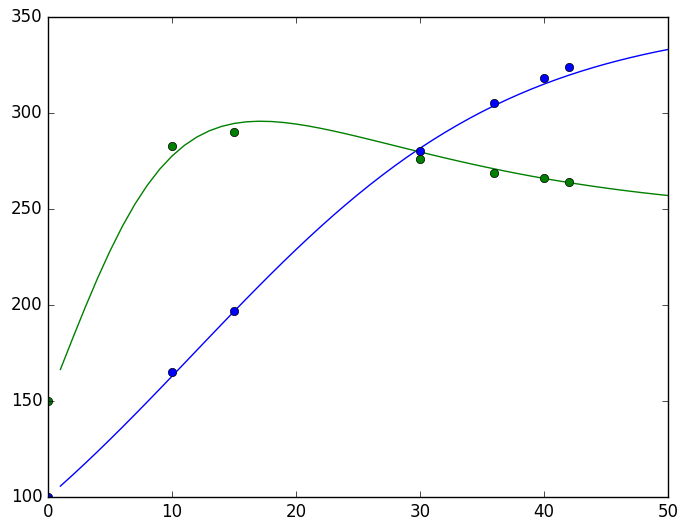RNN做ODE的参数估计效果（散点：有限的实验数据，曲线：估计出来的模型）显然结果是让人满意的。又到总结本文在一个一般的框架下介绍了 RNN 模型及其在 Keras 下的自定义写法，然后揭示了 ODE 与 RNN 的联系。在此基础上，介绍了用 RNN 直接求解 ODE 以及用 RNN 反推 ODE 参数的基本思路。需要提醒读者的是，在 RNN 模型的反向传播中，要谨慎地做好初始化和截断处理处理，并且选择好学习率等，以防止梯度爆炸的出现（梯度消失只是优化得不够好，梯度爆炸则是直接崩溃了，解决梯度爆炸问题尤为重要）。总之，梯度消失和梯度爆炸在 RNN 中是一个很经典的困难，事实上，LSTM、GRU 等模型的引入，根本原因就是为了解决 RNN 的梯度消失问题，而梯度爆炸则是通过使用 tanh 或 sigmoid 激活函数来解决的。但是如果用 RNN 解决 ODE 的话，我们就没有选择激活函数的权利了（激活函数就是 ODE 的一部分），所以只能谨慎地做好初始化及其他处理。据说，只要谨慎做好初始化，普通 RNN 中用 relu 作为激活函数都是无妨的。相关链接. Tian Qi C, Yulia R, Jesse B, David D. Neural Ordinary Differential Equations. arXiv preprint arXiv:1806.07366, 2018.. 两生物种群竞争模型https://kexue.fm/archives/3120声明：文章收集于网络，如有侵权，请联系小编及时处理，谢谢！欢迎加入本站公开兴趣群商业智能与数据分析群兴趣范围包括各种让数据产生价值的办法，实际应用案例分享与讨论，分析工具，ETL工具，数据仓库，数据挖掘工具，报表系统等全方位知识QQ群：81035754

## 热门频道

• 大数据
• 商业智能
• 量化投资
• 科学探索
• 创业GMT+8, 2019-3-23 09:27 , Processed in 0.197448 second(s), 23 queries .